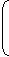# Aptitude - Banker's Discount

Exercise : Banker's Discount - General Questions
6.
The banker's discount of a certain sum of money is Rs. 72 and the true discount on the same sum for the same time is Rs. 60. The sum due is:
Rs. 360
Rs. 432
Rs. 540
Rs. 1080
Explanation:

 Sum = B.D. x T.D. = Rs.72 x 60= Rs.72 x 60= Rs. 360. B.D. - T.D. 72 - 60 12

7.
The certain worth of a certain sum due sometime hence is Rs. 1600 and the true discount is Rs. 160. The banker's gain is:
Rs. 20
Rs. 24
Rs. 16
Rs. 12
Explanation:

 B.G. = (T.D.)2 = Rs.160 x 160= Rs. 16. P.W. 1600

8.
The present worth of a certain bill due sometime hence is Rs. 800 and the true discount is Rs. 36. The banker's discount is:
Rs. 37
Rs. 37.62
Rs. 34.38
Rs. 38.98
Explanation:

 B.G. = (T.D.)2 = Rs.36 x 36= Rs. 1.62 P.W. 800B.D. = (T.D. + B.G.) = Rs. (36 + 1.62) = Rs. 37.62

9.
The banker's gain on a bill due 1 year hence at 12% per annum is Rs. 6. The true discount is:
Rs. 72
Rs. 36
Rs. 54
Rs. 50
Explanation:

 T.D. = B.G. x 100 = Rs.6 x 100= Rs. 50. R x T 12 x 1

10.

 The banker's gain on a certain sum due 1 1 years hence is 3 of the banker's 2 25

discount. The rate percent is:

 5 1 % 5
 9 1 % 11
 8 1 % 8
 6 1 % 6
Explanation:

Let, B.D = Re. 1.

 Then, B.G. = Re. 3 . 25T.D. = (B.D. - B.G.) = Re.1 - 3= Re. 22 . 25 25

 Sum =1 x (22/25)= Rs. 22 . 1-(22/25) 3

 S.I. on Rs. 22 for 1 1 years is Re. 1. 3 2Rate =100 x 1%
 = 100 = 9 1 %. 11 11
 22 x 3 3 2